# Tank 9

The tank with volume V is filled with one pump for three hours and by the second pump for 5 hours. When both pumps run simultaneously, calculate:

a) how much of the total volume of the tank is filled in one hour
b) for how long is the tank full

a =  0
b =  0 h

### Step-by-step explanation:

System of equations are non-linear.
Sorry, equation/equations do not have an integer solution ... Please check them.

Our linear equations calculator calculates it.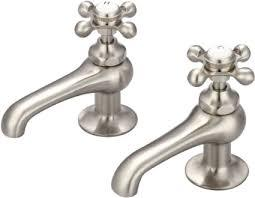Did you find an error or inaccuracy? Feel free to write us. Thank you!Tips to related online calculators
Do you have a linear equation or system of equations and looking for its solution? Or do you have a quadratic equation?
Do you want to convert time units like minutes to seconds?

## Related math problems and questions:

• Three pumps together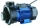One pump fills the tank in 1.5 hours, the second in 2 hours, and the third in 3 hours 20 minutes. How many minutes will the tank fill with three pumps if they work simultaneously?
• Two pumps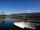The first pump fills the tank in 24 hours second pump in another 40 hours. For how long will it take to fill the tank if it operates both pumps at the same time?
• Three pumpsWe are filling the pool. The first pump would be filled in 12 hours, the second pump in 15 hours. If all three pumps were running at the same time, it would fill the pool for 4 hours. How long would the pool fill only with the third pump?
• Two pumpsPump A takes twice as long to fill a tank as B. Together they take 2 hours to fill the tank. How long does each one take to fill the tank by itself?
• Pumps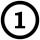First pump flows 16 liters per second into the basin by the second pump 75% of the first and by third pump half more than the second. How long will take fill basin by all three pumps simultaneously volume 15 m3 (cubic meters)?
• Three pumps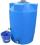The water tank is emptied with the first pump in 6 hours, with the second pump in 2 hours. In order to be emptied in 1 hour, all three pumps had to be started at once. How long will take tank be emptied by only the third pump?
• Two pumps togetherThe first pump will fill the tank itself in 3 hours and the second one in 6 hours. How many hours will the tank be full if both pumps are worked at the same time?
• PumpingThe tank is filled with a larger pump in 12 hours and with scond pump in 15 hours. For how long does the tank fill when both pumps are switched on at the same time?
• Five pumps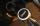Three same pumps fill the tank with 50400 liters of diesel in 7 hours. How many liters of diesel will it take in 4 hours if we add two more of the same pumps and pump them the same way? How much more (or less) will they get if we add 2 of the same pumps a
• Pumps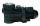The tank is filled with two pumps in 16 minutes. The first pump is filled in 30 minutes earlier than two one. How many minutes is filled with the first pump?
• Pumps A and BPump A fill the tank for 12 minutes, pump B for 24 minutes. How long will take to fill the tank if he is only three minutes works A and then both pumps A and B?
• Water inlets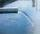Inlet valve with a flow rate of 12 liters per second is filled tank for 72 minutes. How long take to fill full tank if we open one more such valve half an hour after?
• Tank No 8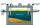Tank is filled by one inlet valve with a flow rate of 12 liters per second in 72 minutes. How long take the tank to fill, if we open half an hour after one more inlet?
• 5 pumps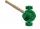Five pump to pump 3 hours 1600 hl of water. How long pumped same amount of water 4 pumps?
• Water pool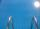Pool with volume 990hl completely filled, if water flows by one tap 8 hours and by second tap 6 hours. First tap give 10hl more than second per hour. How many hl flows in each of them in an hour?
• The dam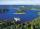The water reservoir is filled with first tributary for 25 hours second tributary for 35 hours. Some time were both inlets open, then a second tributary closed and the tank was filled in four hours. What time water flowed from both tributaries? (Expressed
• Pumps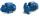Four identical pumps fill the tank in 40 hours. How many pumps would we have to use if we wanted to save 8 hours?